Equations > Calculus > Differentiation/Differentals

Showing results 1 to 10 of 29, on page 1 of 3
Go to page: 1 2 3
 Description Equation Derivative of a Constant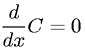Derivative of a Variable to the First Power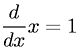Derivative of a Variable to the nth Power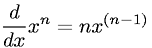Derivative of an Exponential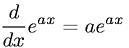Derivative of an Arbitrary Base Exponential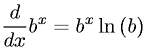Derivative of a Natural LogarithmDerivative of Sine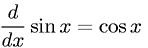Derivative of Cosine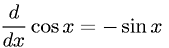Derivative of TangentDerivative of Cotangent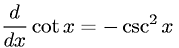(you need to login first) Go to page: 1 2 3